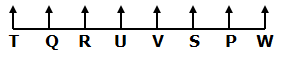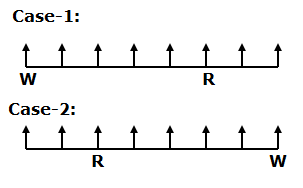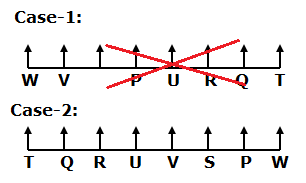# CWC/FCI Prelims 2019 Reasoning Ability Questions (Day-42)

Dear Readers, Exam Race for the Year 2019 has already started, To enrich your preparation here we are providing new series of Practice Questions on CWC/FCI Prelims 2019 Reasoning Ability Questions on daily basis. Aspirants, practice these CWC/FCI Prelims 2019 Reasoning Ability Questions (Day-42) on a regular basis to improve your score in the aptitude section. Start your effective preparation from the right beginning to get success in the upcoming CWC/FCI Exam.

[WpProQuiz 6037]

Direction (1-5): Read the following information carefully and answer the questions given below.

Eight friends P, Q, R, S, T, U, V and W are seated in a straight line and facing north but not necessary in the same order.

W sits at one of the extreme ends of the row. Only four persons are sitting between W and R. Both Q and U are immediate neighbours of R. Only two persons sitting between U and T. T is not an immediate neighbour of W. V sits second to the left of P. P does not sit to the left of T.

1) What is the position of T with respect to R?

a) Third to the right

b) On the immediate left

c) Second to the right

d) Fourth to the right

e) Second to the left

2) Which of the following statements is true?

a) Only three persons are sitting between V and W

b) Only one person sitting to the right of V

c) P sits on the immediate right of V

d) Both T and Q are immediate neighbours of V

e) None is true

3) Who among the following persons sitting at extreme left end of the row?

a) P

b) T

c) V

d) Q

e) S

4) How many persons are sitting between W and P?

a) Two

b) Three

c) None

d) Four

e) One

5) Which of the following pairs sit exactly between U and T?

a) P, V

b) Q, W

c) P, R

d) Q, R

e) S, V

Direction (6-10): These questions are based on five three-digitnumbers given below.

620  537  184  723  463

6) If all the numbers are arranged in descending order from left to right, which of the following will be sum of all three digits of the number which is second from the left?

a) 7

b) 6

c) 8

d) 5

e) None of these

7) What will be the resultant of the product of the first digit of the second highest number and the third digit of the second lowest number?

a) 14

b) 12

c) 16

d) 18

e) 20

8) If the position of second and third digit of each numbers are interchanged. Then, what will be the resultant of the third digit of the lowest number thus formed multiplied by the second digit of the highest number thus formed?

a) 22

b) 24

c) 26

d) 28

e) 18

9) If in each number all the digits are arranged in ascending order from left to right within the number, then how many numbers thus formed will be even number?

a) None

b) One

c) Two

d) Three

e) Four

10) If ‘1’ is subtracted from the second digit of every even number and ‘2’ is added to the third digit of the every odd number. Then in how many numbers will a digit appear twice?

a) Two

b) Three

c) One

d) None

e) Four

Direction (1-5):• W sits at one of the extreme ends of the row. Only four persons are sitting between W and R.• Both Q and U are immediate neighbours of R. Only two persons sitting between U and T. T is not an immediate neighbour of W. V sits second to the left of P. P does not sit to the left of T.
• So, Case-1 will be dropped.Direction (6-10):

620  537  184  723  463

723  620  537  463  184

6+2+0=8.

620  537  184  723  463

6*3=18.

620  537  184  723  463

602  573  148  732  436

8*3=24.

620  537  184  723  463

026  357  148  237  346